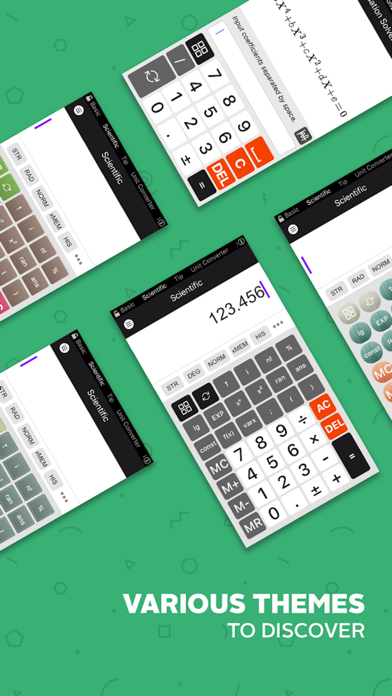# ▸ Calculator +

## iOS iPhoneUtilities

\$1.99

+ 800+ formulas in Formula Toolkit In-App
+ Award Winning Calculator Since 2007

HiCalc Pro is an ultimate calculator and will make all your calculations become simpler than ever with 12 calculators: Basic, Scientific, Currency Converter, Unit Converter, Finance, Date - Time, Constants, Equation Solver, Statistics, Base conversion, Graph & Tip calculator.

► Noteworthy
• Three calculating modes: STANDARD, STRING and RPN
• Precision to 31 digits
• BIG CALC in Portrait mode.
• Comprehensive Date & Time calculator
• Unit Converter contains 3,300 units in 113 categories
• Currency converter with daily online update for 152 currencies
• Extended Memory and Expression History supports
• Intuitive interface
• Export & send out expressions/history

► Features:
• Multi-line LED with smart input for entering complicated expressions
• Show expression as normal writing mode @ very useful for students
• Advanced RPN mode with Stack viewer and functions: DROP, SWAP, ROLL, DEPTH, PICK, OVER, KEEP, LAST, CLEAR
• Sending data from Ext-Memory & History list
• Support 5 languages (English, French, German, Chinese & Vietnamese)

► Scientific calculator:
• Basic fx: sin, cos, tan, sin-1, cos-1, tan-1, sinh, cosh, tanh, log, ln, sinh-1, cosh-1, tanh-1 …
• Advanced fx: Random, nPr, nCr, Differential function, Evaluation function, Integration function, Root-Finder and Product function, Greatest Common Divisor, Least Common Multiple, Fibonacci function …
• Complex numbers in STRING mode

► Unit Converter: a powerful and easy-to-use tool for converting over 3,300 units in 113 categories.
• Special categories: Women and Girl's Clothing, Cooking, Electric Field, Density Traditional, Typography, UHF channel and frequency chart, Fuel Consumption …
• Full text search for categories and units
• Favorite & Recently used units
• Update over the air

► Statistics calculator
• 15 common functions: Σ(x), mean of X, AVD, SSD, SDV, C(n, k), Min, Max, GM...
• 6 probability distribution functions: Binomial, Gamma, Gaussian, Geometric, Poisson, Rayleigh
• Smart data input: data import from clipboard supported

► Graphing Calculator
• Up to three graphs
• Coordinate system movable
• Zoom in/out

► Equation solver
• Polynomial Equations (1st-4th)
• N-simultaneous equations

► Base conversion
• Full-featured base conversion

► Date & Time calculator
• Date / Time difference: calculate duration between two dates.
• Add/ Subtract: Add or subtract days, months and years of a given date to get a new date.
• Events Countdown/up: Shows Time Remaining and Elapsed Time

► World Clock
• Show date & time of any city in the World
• Have 6 cool skins

► Finance calculator
• Time Value of Money (NPER, RATE, PV, FV, PMT)
• Mortgage & Loan Calculator
• Finance functions: SLN, SYD, DB, NPV, IRR, NPER, RATE, PV, FV, PMT.

► Constants Library:
• 1500 constants from Mathematics, Physics, Solar System and Element e.g: Avogadro number, Gravitational const, Stefan-Boltzmann Const, Speed of Light.

## What's New

- Fix bugs on iPhone X and iOS 13

•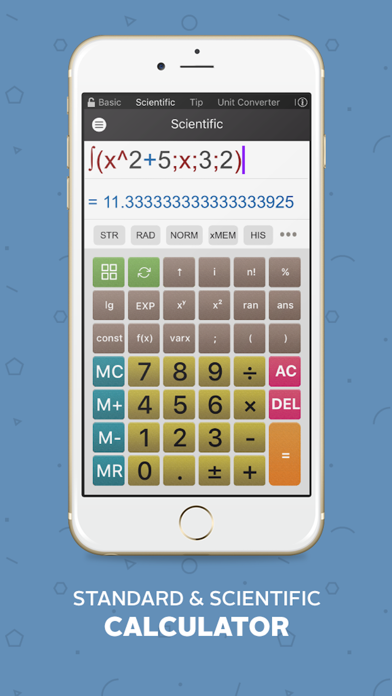•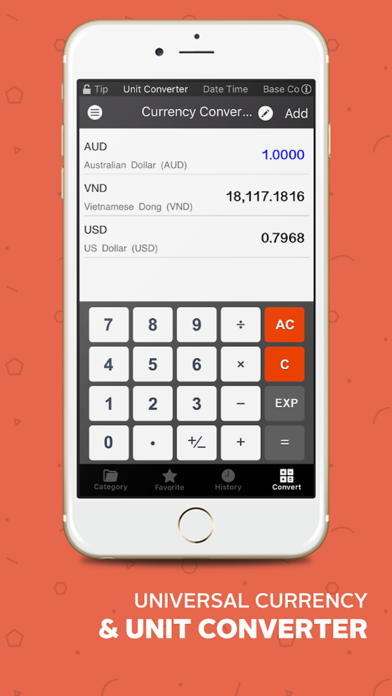•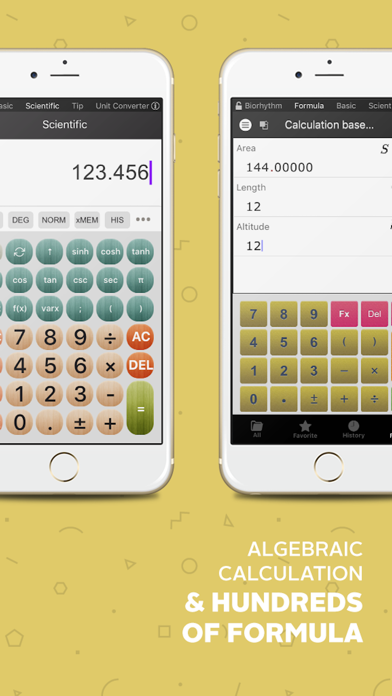•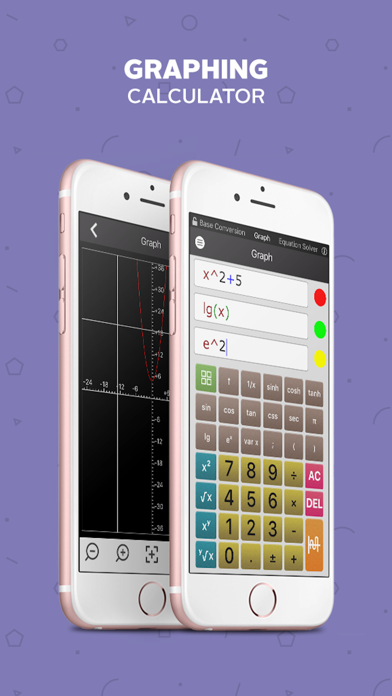•